Data Types in java

View more Tutorials: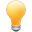Follow us on our fanpages to receive notifications every time there are new articles.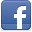Facebook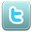Twitter

1- Overview of data types

Any programming language has a set of data types. Data types are basic, and quite similar for all languages. All data types are composed from bits, therefore, I dedicate a post to introduce the history of bits and bytes. My advice is that you should read it before continuing reading this post.
Java has two types of data types:
• Primitive Types
• Reference Types
There are 8 primitive data types which are boolean, byte, char, short, int, long, float, double.
Data type Default Value Size
boolean false 1 bit
char '\u0000' 2 byte
byte 0 1 byte
short 0 2 byte
int 0 4 byte
long 0L 8 byte
float 0.0f 4 byte
double 0.0d 8 byte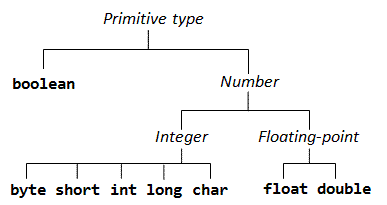• Logic type: boolean.
• Integer types: byte, short, char, int, long.
• Real number type is also called floating point: float, double.
Reference types, which are objects created by the constructor of classes.

2- byte

1 byte a byte is composed of 8 consecutive bits in the memory of computer. Each bit  is a binary number of 0 or 1. Java uses "byte" to name a integer type with small scope (Size: 1 byte).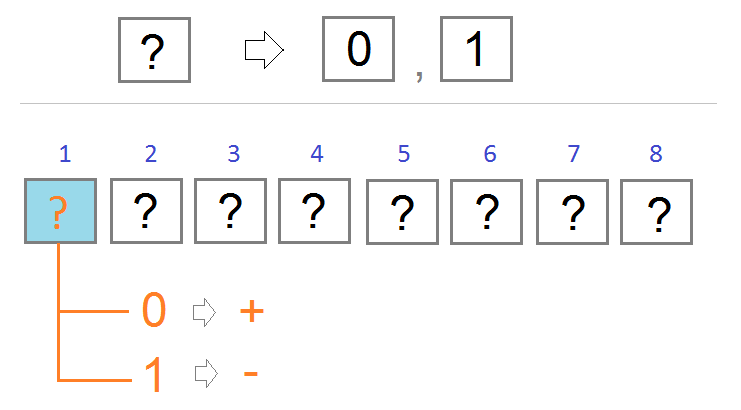The first bit in a row of 8 bits has value of 0 or 1.
• If it is 0, Java considers it as + (Represent a positive number)
• If it is 1 Java considers it as - (Represent a negative number)
For next 7 bits, you can represent a number between 0 and 127. From here you deduce the byte type in Java with the range of [-127, 127].
But wait, it is supposed to be [-128, 127], why?

Why is the smallest number of byte type in Java -128?
If the rules that the first bit has a value of 0 equivalent to +, the value of 1 equivalent to -, then we have two ways to represent 0 (see the illustration).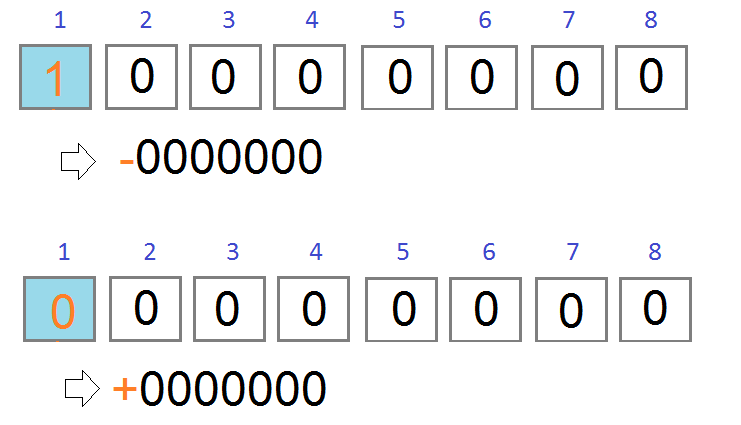Therefore, the binary sequence of "1 0 0 0 0 0 0" should be considered as the smallest number of byte data type in Java. It represents -128.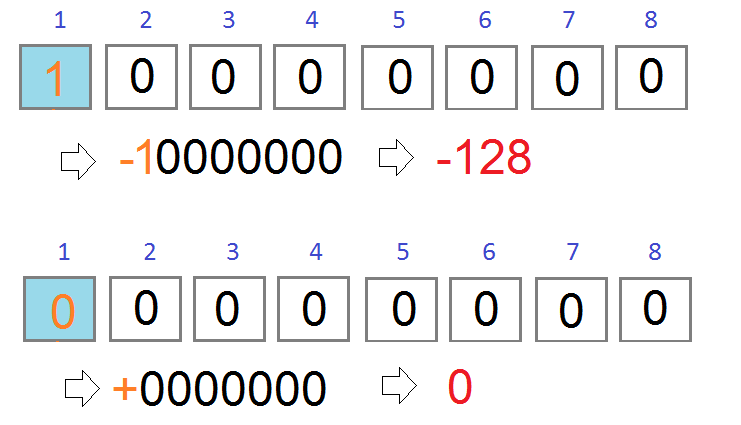3- boolean

boolean is the simplest data type. It has size of 1 bit. It has 2 values of  true and false.
The default value for the boolean type is false.

// Declare a variable named 'valid', data type is boolean
// The value will be false by default.
boolean valid;

// Declare a variable named 'active', data type is boolean
// Value is true.
boolean active = true;

4- char

Although "char" is the first four characters of the "Character" term, the char type in Java is used to store non-negative integers with the two-byte size. It is also used to represent for a Unicode character because in nature, each character corresponds to a specific number. (This number is understood as the code of character.)
Because char is non-negative integer type, size: 2 bytes, its scope is [0, 2*16-1]  ( [0, 65535] ).
When char is understood as a Unicode character, the smallest character is '\u0000' (Mã 0), and the largest character is '\uffff' (code: 65535).
What is Unicode character?
• TODO

5- short

short is data type for the purpose of representing a two-byte integer  (16 bits), including negative integer.
• The smallest value is -32,768 (-2^15) and the largest value is 32,767 (2^15 -1).
• Default value is 0.
** Note: See the explanation of the rule to determine the positive or negative number in the section of byte data type.

6- int

int data type is used to represent an integer with the size of 4 bytes (32 bits).
• The smallest value: -2,147,483,648 (-2^31)
• The largest value:  2,147,483,647 (2^31 -1)
• Default value: 0.
In Java, the int data type is considered as default data type for integers. Therefore, if you write 100, Java will create a four- byte memory area for storage. And if you want Java to create an eight- byte memory area to store 100, you have to write 100L. (long is an eight-byte integer type, introduced in the following section).

int a = 100;

7- long

The long data type is used to represent integers with the size of 8 bytes (64 bits).
• The smallest value is -9,223,372,036,854,775,808.(-2^63)
• The largest value is 9,223,372,036,854,775,807. (2^63 -1)
• This type is used when a value pattern wider than int is necessary.
• Default value is 0L.

// You need to add 'L' immediately after 100
// so that Java creates an 8 byte memory area to store 100
long a = 100L;

8- float

float data type is used to represent a real number with the size of 4 bytes (32 bits).
• The smallest value: -3.4028235 x 10^38
• The largest value:: 3.4028235 x 10^38
• Default value: 0.0f
Example:

float value = 2.5f;

9- double

double data type is used to represent a real number with the size of 8 bytes (64 bits). It is the default type for real numbers.
• The smallest value: -1.7976931348623157 x 10^308
• The largest value:  1.7976931348623157 x 10^308
• Default value: 0.0d
Example:

double a = 2.5d;

// Since doubling is the default type for real numbers,
// You can write more concisely:
double b = 2.5;

10- Reference Types

In Java, a data type created by a combination of primitive types is called a reference type. The most commonly used reference type is the String, which is a combination of characters.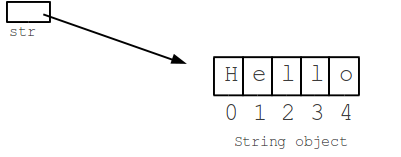Reference types are created based on a class. The class is like a blueprint to define a reference type.

String cityName;
}

class Student {

String fullName;

int age;

}

View more Tutorials:

Maybe you are interested

These are online courses outside the o7planning website that we introduced, which may include free or discounted courses.

•Java Persistence: Hibernate and JPA Fundamentals
•Dart 2 Programming Bootcamp For Complete Beginners
•Learning Path: AWS Certified Developer – Associate Complete
•Master ExpressJS to Build Web Apps with NodeJS&JavaScript
•AWS Lambda and Kinesis in Action
•Twitter Bootstrap 3 for Beginners - With HTML5 and CSS3
•Java Object-Oriented Programming : Build a Quiz Application
•Ready for Production with Spring Boot Actuator
•Flutter & Dart: A Complete Showcase Mobile App™
•MySQL PHP Database Essentials add a Database to your site
•*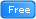* Java Database Connection: JDBC and MySQL
•Creating Reports with SQL Server 2012 Reporting Services
•Build a Chatbot integrated Website using Bootstrap 4
•Learn Android Programming From Scratch - Basics
•Try AngularJS: Beginner's Guide to Front End Web Development
•Happy Flutter - Sport News Apps Flutter
•Basic Concepts of Web Development, HTTP and Java Servlets
•Java JDBC with Oracle: Build a CRUD Application
•Learn C++ by Creating
•JavaScript and React for Developers: Master the Essentials
•Build Responsive Website with HTML5 and CSS3
•HTML5 Mobile Game Development with Phaser
•Complete Step By Step Java For Testers
•Understanding JDBC with PostgreSQL (A step by step guide)